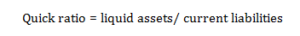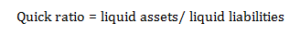# Definition of Quick ratio

One of the important liquidity ratios, quick ratio is used to gauge the short term liquid position of a firm. This ratio establishes a relationship between liquid assets and current liabilities of a company. It is done to find out the efficiency of a business enterprise to pay it’s short term debt obligation.

This ratio is different from current ratio in sense that current ratio takes into consideration, all the current assets of a company while making a comparison with current liabilities. On the other hand, amount of inventory and prepaid expenses (which constitute a major part of current assets) are not taken into consideration while calculating this ratio.

### Important formulas for calculating quick ratio:

This ratio can be calculated by dividing liquid assets by the amount of current liabilities, as shown below:Current liabilities can also be substituted by liquid liabilities. In this case formula will be:This ratio is also known as ‘acid test ratio’ or ‘liquid ratio’.

Posted on Categories Dictionary